Related Articles

# Fleury’s Algorithm for printing Eulerian Path or Circuit

• Difficulty Level : Hard
• Last Updated : 15 Sep, 2021

Eulerian Path is a path in graph that visits every edge exactly once. Eulerian Circuit is an Eulerian Path which starts and ends on the same vertex.
We strongly recommend to first read the following post on Euler Path and Circuit.
https://www.geeksforgeeks.org/eulerian-path-and-circuit/
In the above mentioned post, we discussed the problem of finding out whether a given graph is Eulerian or not. In this post, an algorithm to print Eulerian trail or circuit is discussed.
Following is Fleury’s Algorithm for printing Eulerian trail or cycle (Source Ref1).
1. Make sure the graph has either 0 or 2 odd vertices.
2. If there are 0 odd vertices, start anywhere. If there are 2 odd vertices, start at one of them.
3. Follow edges one at a time. If you have a choice between a bridge and a non-bridge, always choose the non-bridge.
4. Stop when you run out of edges.
The idea is, “don’t burn bridges so that we can come back to a vertex and traverse remaining edges. For example let us consider the following graph.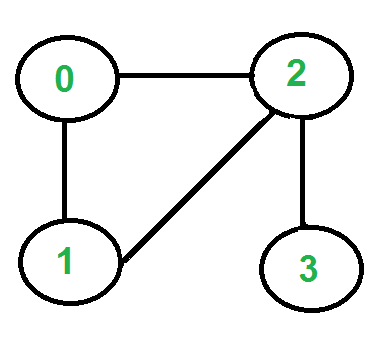There are two vertices with odd degree, ‘2’ and ‘3’, we can start path from any of them. Let us start tour from vertex ‘2’.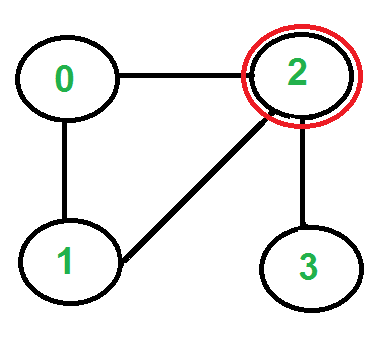There are three edges going out from vertex ‘2’, which one to pick? We don’t pick the edge ‘2-3’ because that is a bridge (we won’t be able to come back to ‘3’). We can pick any of the remaining two edge. Let us say we pick ‘2-0’. We remove this edge and move to vertex ‘0’.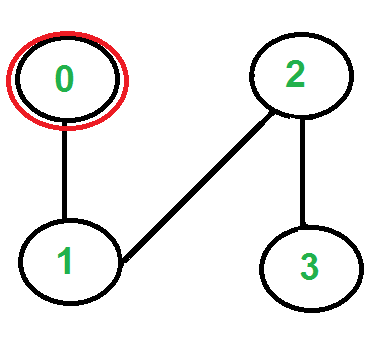There is only one edge from vertex ‘0’, so we pick it, remove it and move to vertex ‘1’. Euler tour becomes ‘2-0 0-1’.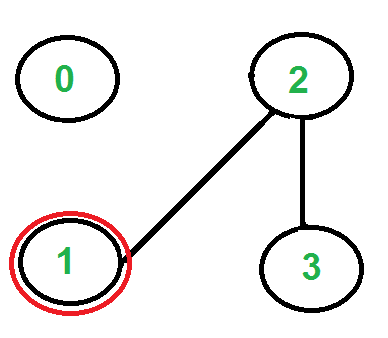There is only one edge from vertex ‘1’, so we pick it, remove it and move to vertex ‘2’. Euler tour becomes ‘2-0 0-1 1-2’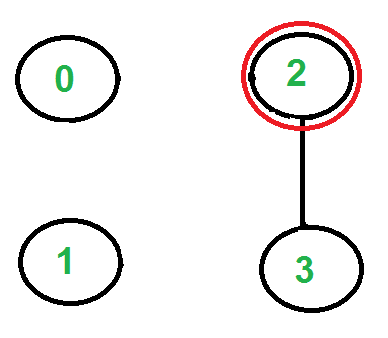Again there is only one edge from vertex 2, so we pick it, remove it and move to vertex 3. Euler tour becomes ‘2-0 0-1 1-2 2-3’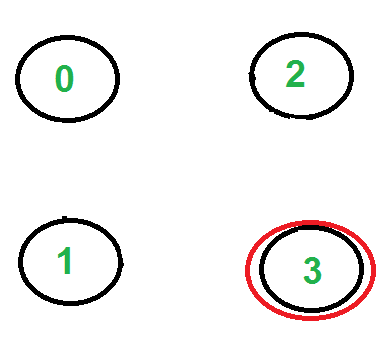There are no more edges left, so we stop here. Final tour is ‘2-0 0-1 1-2 2-3’.
See this for and this fore more examples.
Following is C++ implementation of above algorithm. In the following code, it is assumed that the given graph has an Eulerian trail or Circuit. The main focus is to print an Eulerian trail or circuit. We can use isEulerian() to first check whether there is an Eulerian Trail or Circuit in the given graph.
We first find the starting point which must be an odd vertex (if there are odd vertices) and store it in variable ‘u’. If there are zero odd vertices, we start from vertex ‘0’. We call printEulerUtil() to print Euler tour starting with u. We traverse all adjacent vertices of u, if there is only one adjacent vertex, we immediately consider it. If there are more than one adjacent vertices, we consider an adjacent v only if edge u-v is not a bridge. How to find if a given is edge is bridge? We count number of vertices reachable from u. We remove edge u-v and again count number of reachable vertices from u. If number of reachable vertices are reduced, then edge u-v is a bridge. To count reachable vertices, we can either use BFS or DFS, we have used DFS in the above code. The function DFSCount(u) returns number of vertices reachable from u.
Once an edge is processed (included in Euler tour), we remove it from the graph. To remove the edge, we replace the vertex entry with -1 in adjacency list. Note that simply deleting the node may not work as the code is recursive and a parent call may be in middle of adjacency list.

## C++

 `// A C++ program print Eulerian Trail in a given Eulerian or``// Semi-Eulerian Graph``#include ``#include ``#include ``#include ``using` `namespace` `std;` `// A class that represents an undirected graph``class` `Graph {``    ``int` `V; ``// No. of vertices``    ``list<``int``>* adj; ``// A dynamic array of adjacency lists``public``:``    ``// Constructor and destructor``    ``Graph(``int` `V)``    ``{``        ``this``->V = V;``        ``adj = ``new` `list<``int``>[V];``    ``}``    ``~Graph() { ``delete``[] adj; }` `    ``// functions to add and remove edge``    ``void` `addEdge(``int` `u, ``int` `v)``    ``{``        ``adj[u].push_back(v);``        ``adj[v].push_back(u);``    ``}``    ``void` `rmvEdge(``int` `u, ``int` `v);` `    ``// Methods to print Eulerian tour``    ``void` `printEulerTour();``    ``void` `printEulerUtil(``int` `s);` `    ``// This function returns count of vertices reachable``    ``// from v. It does DFS``    ``int` `DFSCount(``int` `v, ``bool` `visited[]);` `    ``// Utility function to check if edge u-v is a valid next``    ``// edge in Eulerian trail or circuit``    ``bool` `isValidNextEdge(``int` `u, ``int` `v);``};` `/* The main function that print Eulerian Trail. It first``   ``finds an odd degree vertex (if there is any) and then``   ``calls printEulerUtil() to print the path */``void` `Graph::printEulerTour()``{``    ``// Find a vertex with odd degree``    ``int` `u = 0;``    ``for` `(``int` `i = 0; i < V; i++)``        ``if` `(adj[i].size() & 1) {``            ``u = i;``            ``break``;``        ``}` `    ``// Print tour starting from oddv``    ``printEulerUtil(u);``    ``cout << endl;``}` `// Print Euler tour starting from vertex u``void` `Graph::printEulerUtil(``int` `u)``{``    ``// Recur for all the vertices adjacent to this vertex``    ``list<``int``>::iterator i;``    ``for` `(i = adj[u].begin(); i != adj[u].end(); ++i) {``        ``int` `v = *i;` `        ``// If edge u-v is not removed and it's a a valid``        ``// next edge``        ``if` `(v != -1 && isValidNextEdge(u, v)) {``            ``cout << u << ``"-"` `<< v << ``"  "``;``            ``rmvEdge(u, v);``            ``printEulerUtil(v);``        ``}``    ``}``}` `// The function to check if edge u-v can be considered as``// next edge in Euler Tout``bool` `Graph::isValidNextEdge(``int` `u, ``int` `v)``{``    ``// The edge u-v is valid in one of the following two``    ``// cases:` `    ``// 1) If v is the only adjacent vertex of u``    ``int` `count = 0; ``// To store count of adjacent vertices``    ``list<``int``>::iterator i;``    ``for` `(i = adj[u].begin(); i != adj[u].end(); ++i)``        ``if` `(*i != -1)``            ``count++;``    ``if` `(count == 1)``        ``return` `true``;` `    ``// 2) If there are multiple adjacents, then u-v is not a``    ``// bridge Do following steps to check if u-v is a bridge` `    ``// 2.a) count of vertices reachable from u``    ``bool` `visited[V];``    ``memset``(visited, ``false``, V);``    ``int` `count1 = DFSCount(u, visited);` `    ``// 2.b) Remove edge (u, v) and after removing the edge,``    ``// count vertices reachable from u``    ``rmvEdge(u, v);``    ``memset``(visited, ``false``, V);``    ``int` `count2 = DFSCount(u, visited);` `    ``// 2.c) Add the edge back to the graph``    ``addEdge(u, v);` `    ``// 2.d) If count1 is greater, then edge (u, v) is a``    ``// bridge``    ``return` `(count1 > count2) ? ``false` `: ``true``;``}` `// This function removes edge u-v from graph.  It removes``// the edge by replacing adjacent vertex value with -1.``void` `Graph::rmvEdge(``int` `u, ``int` `v)``{``    ``// Find v in adjacency list of u and replace it with -1``    ``list<``int``>::iterator iv``        ``= find(adj[u].begin(), adj[u].end(), v);``    ``*iv = -1;` `    ``// Find u in adjacency list of v and replace it with -1``    ``list<``int``>::iterator iu``        ``= find(adj[v].begin(), adj[v].end(), u);``    ``*iu = -1;``}` `// A DFS based function to count reachable vertices from v``int` `Graph::DFSCount(``int` `v, ``bool` `visited[])``{``    ``// Mark the current node as visited``    ``visited[v] = ``true``;``    ``int` `count = 1;` `    ``// Recur for all vertices adjacent to this vertex``    ``list<``int``>::iterator i;``    ``for` `(i = adj[v].begin(); i != adj[v].end(); ++i)``        ``if` `(*i != -1 && !visited[*i])``            ``count += DFSCount(*i, visited);` `    ``return` `count;``}` `// Driver program to test above function``int` `main()``{``    ``// Let us first create and test graphs shown in above``    ``// figure``    ``Graph g1(4);``    ``g1.addEdge(0, 1);``    ``g1.addEdge(0, 2);``    ``g1.addEdge(1, 2);``    ``g1.addEdge(2, 3);``    ``g1.printEulerTour();` `    ``Graph g2(3);``    ``g2.addEdge(0, 1);``    ``g2.addEdge(1, 2);``    ``g2.addEdge(2, 0);``    ``g2.printEulerTour();` `    ``Graph g3(5);``    ``g3.addEdge(1, 0);``    ``g3.addEdge(0, 2);``    ``g3.addEdge(2, 1);``    ``g3.addEdge(0, 3);``    ``g3.addEdge(3, 4);``    ``g3.addEdge(3, 2);``    ``g3.addEdge(3, 1);``    ``g3.addEdge(2, 4);``    ``g3.printEulerTour();` `    ``return` `0;``}`

## Java

 `// A Java program print Eulerian Trail``// in a given Eulerian or Semi-Eulerian Graph``import` `java.util.ArrayList;` `// An Undirected graph using``// adjacency list representation``public` `class` `Graph {` `    ``private` `int` `vertices; ``// No. of vertices``    ``private` `ArrayList[] adj; ``// adjacency list` `    ``// Constructor``    ``Graph(``int` `numOfVertices)``    ``{``        ``// initialise vertex count``        ``this``.vertices = numOfVertices;` `        ``// initialise adjacency list``        ``initGraph();``    ``}` `    ``// utility method to initialise adjacency list``    ``@SuppressWarnings``(``"unchecked"``) ``private` `void` `initGraph()``    ``{``        ``adj = ``new` `ArrayList[vertices];``        ``for` `(``int` `i = ``0``; i < vertices; i++) {``            ``adj[i] = ``new` `ArrayList<>();``        ``}``    ``}` `    ``// add edge u-v``    ``private` `void` `addEdge(Integer u, Integer v)``    ``{``        ``adj[u].add(v);``        ``adj[v].add(u);``    ``}` `    ``// This function removes edge u-v from graph.``    ``private` `void` `removeEdge(Integer u, Integer v)``    ``{``        ``adj[u].remove(v);``        ``adj[v].remove(u);``    ``}` `    ``/* The main function that print Eulerian Trail.``       ``It first finds an odd degree vertex (if there``       ``is any) and then calls printEulerUtil() to``       ``print the path */``    ``private` `void` `printEulerTour()``    ``{``        ``// Find a vertex with odd degree``        ``Integer u = ``0``;``        ``for` `(``int` `i = ``0``; i < vertices; i++) {``            ``if` `(adj[i].size() % ``2` `== ``1``) {``                ``u = i;``                ``break``;``            ``}``        ``}` `        ``// Print tour starting from oddv``        ``printEulerUtil(u);``        ``System.out.println();``    ``}` `    ``// Print Euler tour starting from vertex u``    ``private` `void` `printEulerUtil(Integer u)``    ``{``        ``// Recur for all the vertices adjacent to this``        ``// vertex``        ``for` `(``int` `i = ``0``; i < adj[u].size(); i++) {``            ``Integer v = adj[u].get(i);``            ``// If edge u-v is a valid next edge``            ``if` `(isValidNextEdge(u, v)) {``                ``System.out.print(u + ``"-"` `+ v + ``" "``);` `                ``// This edge is used so remove it now``                ``removeEdge(u, v);``                ``printEulerUtil(v);``            ``}``        ``}``    ``}` `    ``// The function to check if edge u-v can be``    ``// considered as next edge in Euler Tout``    ``private` `boolean` `isValidNextEdge(Integer u, Integer v)``    ``{``        ``// The edge u-v is valid in one of the``        ``// following two cases:` `        ``// 1) If v is the only adjacent vertex of u``        ``// ie size of adjacent vertex list is 1``        ``if` `(adj[u].size() == ``1``) {``            ``return` `true``;``        ``}` `        ``// 2) If there are multiple adjacents, then``        ``// u-v is not a bridge Do following steps``        ``// to check if u-v is a bridge``        ``// 2.a) count of vertices reachable from u``        ``boolean``[] isVisited = ``new` `boolean``[``this``.vertices];``        ``int` `count1 = dfsCount(u, isVisited);` `        ``// 2.b) Remove edge (u, v) and after removing``        ``//  the edge, count vertices reachable from u``        ``removeEdge(u, v);``        ``isVisited = ``new` `boolean``[``this``.vertices];``        ``int` `count2 = dfsCount(u, isVisited);` `        ``// 2.c) Add the edge back to the graph``        ``addEdge(u, v);``        ``return` `(count1 > count2) ? ``false` `: ``true``;``    ``}` `    ``// A DFS based function to count reachable``    ``// vertices from v``    ``private` `int` `dfsCount(Integer v, ``boolean``[] isVisited)``    ``{``        ``// Mark the current node as visited``        ``isVisited[v] = ``true``;``        ``int` `count = ``1``;``        ``// Recur for all vertices adjacent to this vertex``        ``for` `(``int` `adj : adj[v]) {``            ``if` `(!isVisited[adj]) {``                ``count = count + dfsCount(adj, isVisited);``            ``}``        ``}``        ``return` `count;``    ``}` `    ``// Driver program to test above function``    ``public` `static` `void` `main(String a[])``    ``{``        ``// Let us first create and test``        ``// graphs shown in above figure``        ``Graph g1 = ``new` `Graph(``4``);``        ``g1.addEdge(``0``, ``1``);``        ``g1.addEdge(``0``, ``2``);``        ``g1.addEdge(``1``, ``2``);``        ``g1.addEdge(``2``, ``3``);``        ``g1.printEulerTour();` `        ``Graph g2 = ``new` `Graph(``3``);``        ``g2.addEdge(``0``, ``1``);``        ``g2.addEdge(``1``, ``2``);``        ``g2.addEdge(``2``, ``0``);``        ``g2.printEulerTour();` `        ``Graph g3 = ``new` `Graph(``5``);``        ``g3.addEdge(``1``, ``0``);``        ``g3.addEdge(``0``, ``2``);``        ``g3.addEdge(``2``, ``1``);``        ``g3.addEdge(``0``, ``3``);``        ``g3.addEdge(``3``, ``4``);``        ``g3.addEdge(``3``, ``2``);``        ``g3.addEdge(``3``, ``1``);``        ``g3.addEdge(``2``, ``4``);``        ``g3.printEulerTour();``    ``}``}` `// This code is contributed by Himanshu Shekhar`

## Python

 `# Python program print Eulerian Trail in a given Eulerian or Semi-Eulerian Graph`` ` `from` `collections ``import` `defaultdict`` ` `#This class represents an undirected graph using adjacency list representation``class` `Graph:`` ` `    ``def` `__init__(``self``,vertices):``        ``self``.V``=` `vertices ``#No. of vertices``        ``self``.graph ``=` `defaultdict(``list``) ``# default dictionary to store graph``        ``self``.Time ``=` `0`` ` `    ``# function to add an edge to graph``    ``def` `addEdge(``self``,u,v):``        ``self``.graph[u].append(v)``        ``self``.graph[v].append(u)` `    ``# This function removes edge u-v from graph   ``    ``def` `rmvEdge(``self``, u, v):``        ``for` `index, key ``in` `enumerate``(``self``.graph[u]):``            ``if` `key ``=``=` `v:``                ``self``.graph[u].pop(index)``        ``for` `index, key ``in` `enumerate``(``self``.graph[v]):``            ``if` `key ``=``=` `u:``                ``self``.graph[v].pop(index)` `    ``# A DFS based function to count reachable vertices from v``    ``def` `DFSCount(``self``, v, visited):``        ``count ``=` `1``        ``visited[v] ``=` `True``        ``for` `i ``in` `self``.graph[v]:``            ``if` `visited[i] ``=``=` `False``:``                ``count ``=` `count ``+` `self``.DFSCount(i, visited)        ``        ``return` `count` `    ``# The function to check if edge u-v can be considered as next edge in``    ``# Euler Tour``    ``def` `isValidNextEdge(``self``, u, v):``        ``# The edge u-v is valid in one of the following two cases:`` ` `          ``#  1) If v is the only adjacent vertex of u``        ``if` `len``(``self``.graph[u]) ``=``=` `1``:``            ``return` `True``        ``else``:``            ``'''``             ``2) If there are multiple adjacents, then u-v is not a bridge``                 ``Do following steps to check if u-v is a bridge`` ` `            ``2.a) count of vertices reachable from u'''`   `            ``visited ``=``[``False``]``*``(``self``.V)``            ``count1 ``=` `self``.DFSCount(u, visited)` `            ``'''2.b) Remove edge (u, v) and after removing the edge, count``                ``vertices reachable from u'''``            ``self``.rmvEdge(u, v)``            ``visited ``=``[``False``]``*``(``self``.V)``            ``count2 ``=` `self``.DFSCount(u, visited)` `            ``#2.c) Add the edge back to the graph``            ``self``.addEdge(u,v)` `            ``# 2.d) If count1 is greater, then edge (u, v) is a bridge``            ``return` `False` `if` `count1 > count2 ``else` `True`  `    ``# Print Euler tour starting from vertex u``    ``def` `printEulerUtil(``self``, u):``        ``#Recur for all the vertices adjacent to this vertex``        ``for` `v ``in` `self``.graph[u]:``            ``#If edge u-v is not removed and it's a a valid next edge``            ``if` `self``.isValidNextEdge(u, v):``                ``print``(``"%d-%d "` `%``(u,v)),``                ``self``.rmvEdge(u, v)``                ``self``.printEulerUtil(v)`  `    ` `    ``'''The main function that print Eulerian Trail. It first finds an odd``   ``degree vertex (if there is any) and then calls printEulerUtil()``   ``to print the path '''``    ``def` `printEulerTour(``self``):``        ``#Find a vertex with odd degree``        ``u ``=` `0``        ``for` `i ``in` `range``(``self``.V):``            ``if` `len``(``self``.graph[i]) ``%``2` `!``=` `0` `:``                ``u ``=` `i``                ``break``        ``# Print tour starting from odd vertex``        ``print` `(``"\n"``)``        ``self``.printEulerUtil(u)` `# Create a graph given in the above diagram` `g1 ``=` `Graph(``4``)``g1.addEdge(``0``, ``1``)``g1.addEdge(``0``, ``2``)``g1.addEdge(``1``, ``2``)``g1.addEdge(``2``, ``3``)``g1.printEulerTour()`  `g2 ``=` `Graph(``3``)``g2.addEdge(``0``, ``1``)``g2.addEdge(``1``, ``2``)``g2.addEdge(``2``, ``0``)``g2.printEulerTour()` `g3 ``=` `Graph (``5``)``g3.addEdge(``1``, ``0``)``g3.addEdge(``0``, ``2``)``g3.addEdge(``2``, ``1``)``g3.addEdge(``0``, ``3``)``g3.addEdge(``3``, ``4``)``g3.addEdge(``3``, ``2``)``g3.addEdge(``3``, ``1``)``g3.addEdge(``2``, ``4``)``g3.printEulerTour()`  `#This code is contributed by Neelam Yadav`

## C#

 `// A C# program print Eulerian Trail``// in a given Eulerian or Semi-Eulerian Graph``using` `System;``using` `System.Collections.Generic;` `// An Undirected graph using``// adjacency list representation``class` `Graph``{``    ``private` `int` `vertices; ``// No. of vertices``    ``private` `List<``int``>[] adj; ``// adjacency list` `    ``// Constructor``    ``Graph(``int` `numOfVertices)``    ``{``        ``// initialise vertex count``        ``this``.vertices = numOfVertices;` `        ``// initialise adjacency list``        ``initGraph();``    ``}` `    ``// utility method to initialise adjacency list``    ``private` `void` `initGraph()``    ``{``        ``adj = ``new` `List<``int``>[vertices];``        ``for` `(``int` `i = 0; i < vertices; i++)``        ``{``            ``adj[i] = ``new` `List<``int``>();``        ``}``    ``}` `    ``// add edge u-v``    ``private` `void` `addEdge(``int` `u, ``int` `v)``    ``{``        ``adj[u].Add(v);``        ``adj[v].Add(u);``    ``}` `    ``// This function removes edge u-v from graph.``    ``private` `void` `removeEdge(``int` `u, ``int` `v)``    ``{``        ``adj[u].Remove(v);``        ``adj[v].Remove(u);``    ``}` `    ``/* The main function that print Eulerian Trail.``    ``It first finds an odd degree vertex (if there``    ``is any) and then calls printEulerUtil() to``    ``print the path */``    ``private` `void` `printEulerTour()``    ``{``        ``// Find a vertex with odd degree``        ``int` `u = 0;``        ``for` `(``int` `i = 0; i < vertices; i++)``        ``{``            ``if` `(adj[i].Count % 2 == 1)``            ``{``                ``u = i;``                ``break``;``            ``}``        ``}``        ` `        ``// Print tour starting from oddv``        ``printEulerUtil(u);``        ``Console.WriteLine();``    ``}` `    ``// Print Euler tour starting from vertex u``    ``private` `void` `printEulerUtil(``int` `u)``    ``{``        ``// Recur for all the vertices``        ``// adjacent to this vertex``        ``for` `(``int` `i = 0; i < adj[u].Count; i++)``        ``{``            ``int` `v = adj[u][i];``            ` `            ``// If edge u-v is a valid next edge``            ``if` `(isValidNextEdge(u, v))``            ``{``                ``Console.Write(u + ``"-"` `+ v + ``" "``);``                ` `                ``// This edge is used so remove it now``                ``removeEdge(u, v);``                ``printEulerUtil(v);``            ``}``        ``}``    ``}` `    ``// The function to check if edge u-v can be``    ``// considered as next edge in Euler Tout``    ``private` `bool` `isValidNextEdge(``int` `u, ``int` `v)``    ``{``        ``// The edge u-v is valid in one of the``        ``// following two cases:` `        ``// 1) If v is the only adjacent vertex of u``        ``// ie size of adjacent vertex list is 1``        ``if` `(adj[u].Count == 1)``        ``{``            ``return` `true``;``        ``}` `        ``// 2) If there are multiple adjacents, then``        ``// u-v is not a bridge Do following steps``        ``// to check if u-v is a bridge``        ``// 2.a) count of vertices reachable from u``        ``bool``[] isVisited = ``new` `bool``[``this``.vertices];``        ``int` `count1 = dfsCount(u, isVisited);` `        ``// 2.b) Remove edge (u, v) and after removing``        ``// the edge, count vertices reachable from u``        ``removeEdge(u, v);``        ``isVisited = ``new` `bool``[``this``.vertices];``        ``int` `count2 = dfsCount(u, isVisited);` `        ``// 2.c) Add the edge back to the graph``        ``addEdge(u, v);``        ``return` `(count1 > count2) ? ``false` `: ``true``;``    ``}` `    ``// A DFS based function to count reachable``    ``// vertices from v``    ``private` `int` `dfsCount(``int` `v, ``bool``[] isVisited)``    ``{``        ``// Mark the current node as visited``        ``isVisited[v] = ``true``;``        ``int` `count = 1;``        ` `        ``// Recur for all vertices adjacent``        ``// to this vertex``        ``foreach``(``int` `i ``in` `adj[v])``        ``{``            ``if` `(!isVisited[i])``            ``{``                ``count = count + dfsCount(i, isVisited);``            ``}``        ``}``        ``return` `count;``    ``}` `    ``// Driver Code``    ``public` `static` `void` `Main(String []a)``    ``{``        ``// Let us first create and test``        ``// graphs shown in above figure``        ``Graph g1 = ``new` `Graph(4);``        ``g1.addEdge(0, 1);``        ``g1.addEdge(0, 2);``        ``g1.addEdge(1, 2);``        ``g1.addEdge(2, 3);``        ``g1.printEulerTour();` `        ``Graph g2 = ``new` `Graph(3);``        ``g2.addEdge(0, 1);``        ``g2.addEdge(1, 2);``        ``g2.addEdge(2, 0);``        ``g2.printEulerTour();` `        ``Graph g3 = ``new` `Graph(5);``        ``g3.addEdge(1, 0);``        ``g3.addEdge(0, 2);``        ``g3.addEdge(2, 1);``        ``g3.addEdge(0, 3);``        ``g3.addEdge(3, 4);``        ``g3.addEdge(3, 2);``        ``g3.addEdge(3, 1);``        ``g3.addEdge(2, 4);``        ``g3.printEulerTour();``    ``}``}` `// This code is contributed by PrinciRaj1992`

Output:

```2-0  0-1  1-2  2-3
0-1  1-2  2-0
0-1  1-2  2-0  0-3  3-4  4-2  2-3  3-1```

Note that the above code modifies given graph, we can create a copy of graph if we don’t want the given graph to be modified.
Time Complexity: Time complexity of the above implementation is O ((V+E)2). The function printEulerUtil() is like DFS and it calls isValidNextEdge() which also does DFS two times. Time complexity of DFS for adjacency list representation is O(V+E). Therefore overall time complexity is O((V+E)*(V+E)) which can be written as O(E2) for a connected graph.
There are better algorithms to print Euler tour, Hierholzer’s Algorithm finds in O(V+E) time.
References:
http://www.math.ku.edu/~jmartin/courses/math105-F11/Lectures/chapter5-part2.pdf
http://en.wikipedia.org/wiki/Eulerian_path#Fleury.27s_algorithm